# Volume of Right Prisms

In this worksheet students will learn the formula for finding the volume of various right prisms and apply them to find the volume.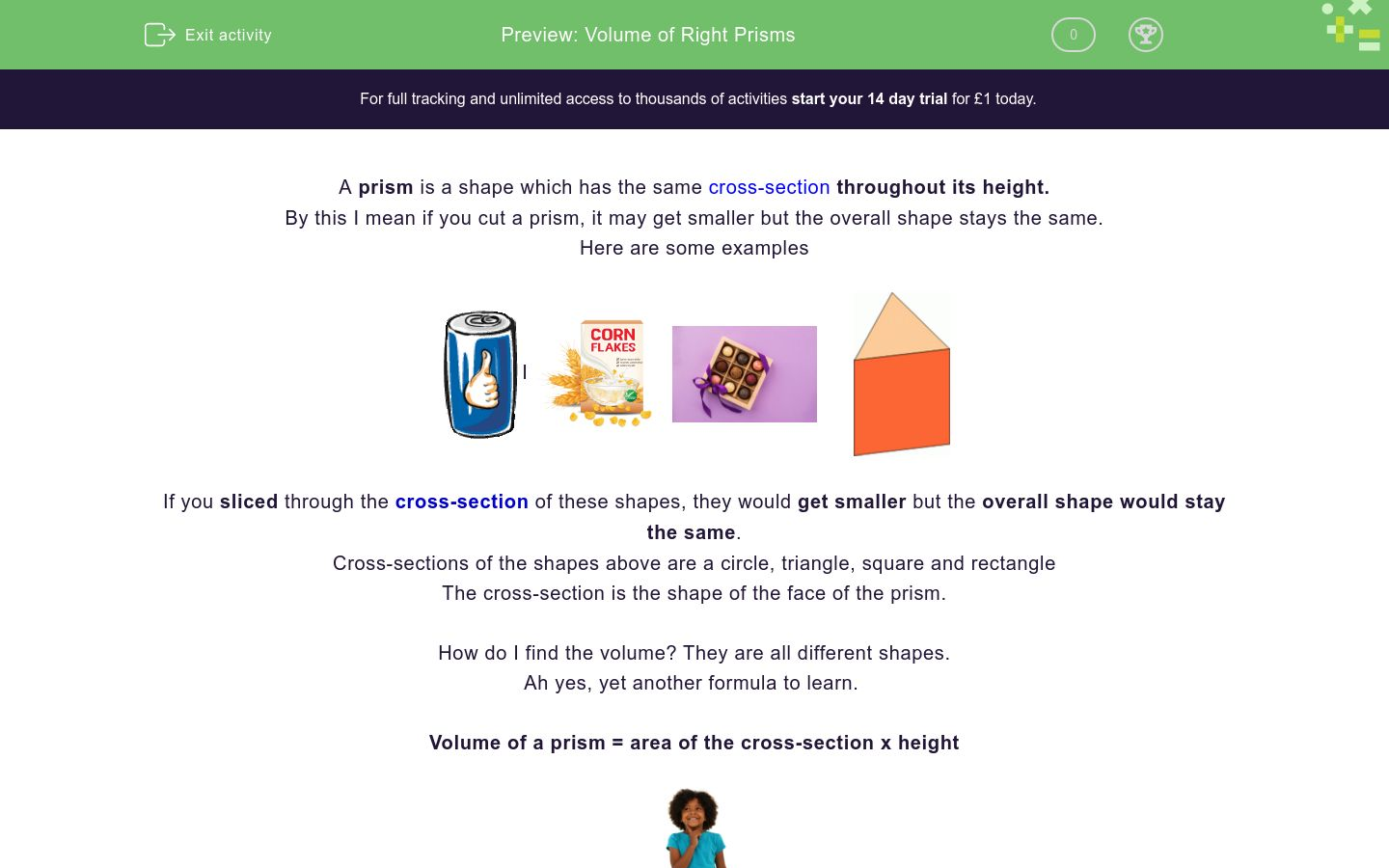Key stage:  KS 4

GCSE Subjects:   Maths

GCSE Boards:   AQA, Eduqas, Pearson Edexcel, OCR

Curriculum topic:   Geometry and Measures, Mensuration

Curriculum subtopic:   Mensuration and Calculation, Volume and Surface Area Calculations

Difficulty level:### QUESTION 1 of 10

A prism is a shape which has the same cross-section throughout its height.

By this I mean if you cut a prism, it may get smaller but the overall shape stays the same.

Here are some examplesIIf you sliced through the cross-section of these shapes, they would get smaller but the overall shape would stay the same.

Cross-sections of the shapes above are a circle, triangle, square and rectangle

The cross-section is the shape of the face of the prism.

How do I find the volume? They are all different shapes.

Ah yes, yet another formula to learn.

Volume of a prism = area of the cross-section x heightBefore we continue we need a recap of how to find the area of these shapes.Area of a square = base x heightArea of a rectangle = base x height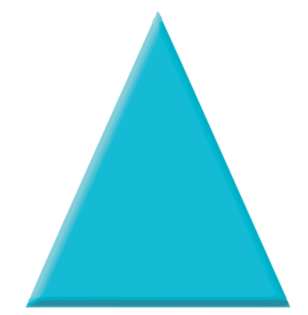Area of a triangle = base x height ÷ 2Area of a circle =  π x r x r

Can you remember the value of π?  Don't worry you will find out later if you have forgotten.

Almost there.  To find the volume we calculate the area of the face first and then multiply by the height.Time to practice.Find the volume of this box of chocolates. Base = 6 cm.

The face  (cross-section) is a square - find the area first.

6 x 6 = 36 cm, now just multiply by the length, which as its a square box  is also 6 cm.   36 x 6 = 216 cm³Find the volume of the cornflakes box.  Base 12 cm, height 6 cm, length 30 cm

The face  (cross-section) is a rectangle - find the area first.

12 x 6 = 72 cm, now just multiply by the length   72 x 30 = 2160 cm³Find the volume of the triangular prism.  Base 4 cm, height 4 cm, length 12 cm

The face  (cross-section) is a triangle - find the area first.

4 x 4 = 16 ÷ 2; = 8 cm, now just multiply by the length 8 x 12 = 96 cm³Find the volume of this can.  Radius 4 cm, length 8 cm.

The face  (cross-section) is a circle - find the area first.

Did you remember the value of π, of course, 3.142

Area = 3.142 x 4 x 4 = 50.27

Now multiply by length 50.27 x 8 = 402.16 cm³

That was a bit of a marathon, wasn't it?  Guess what, it is not over...

Activity time!The length of this cylinder is 12 cm.  The radius of the face is 5 cm.

What is the volume of the cylinder?

60 cm³

942.6 cm³

300 cm³

745.4 cm³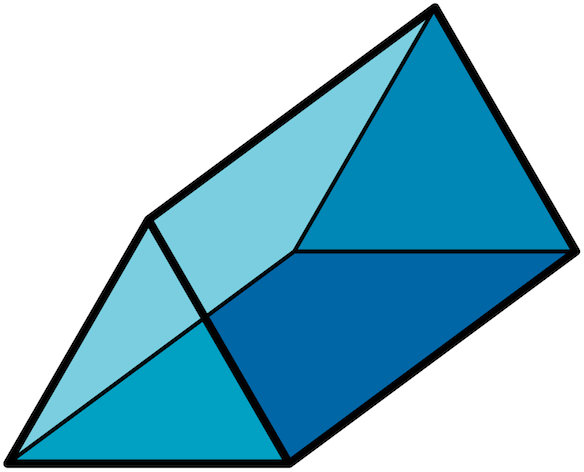What is the volume of this triangular prism.

Base is 6 cm, height 7 cm and length 9 cm.

60 cm³

942.6 cm³

300 cm³

745.4 cm³

Can you work out the following?

## Column B

Cube side 8 cm
512 cm³
Triangular prism base 3cm, height 6 cm, length 8 c...
72 cm³
Cylinder length 12 cm, radius 5 cm
720 cm³
Cereal box base 12 cm, width 4 cm, length 15 cm
942.6 cm³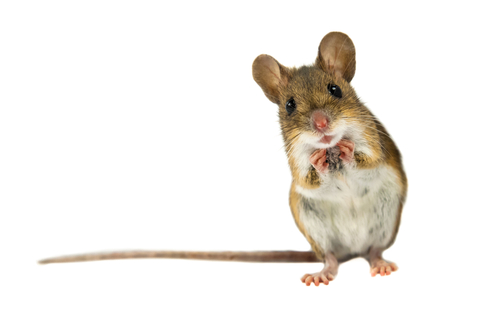This mouse has started eating this wedge of cheese.

The dimensions of the cheese are base 7 cm, height 8 cm and height 8 cm.

What is the volume of cheese the mouse has to get through?

## Column B

Cube side 8 cm
512 cm³
Triangular prism base 3cm, height 6 cm, length 8 c...
72 cm³
Cylinder length 12 cm, radius 5 cm
720 cm³
Cereal box base 12 cm, width 4 cm, length 15 cm
942.6 cm³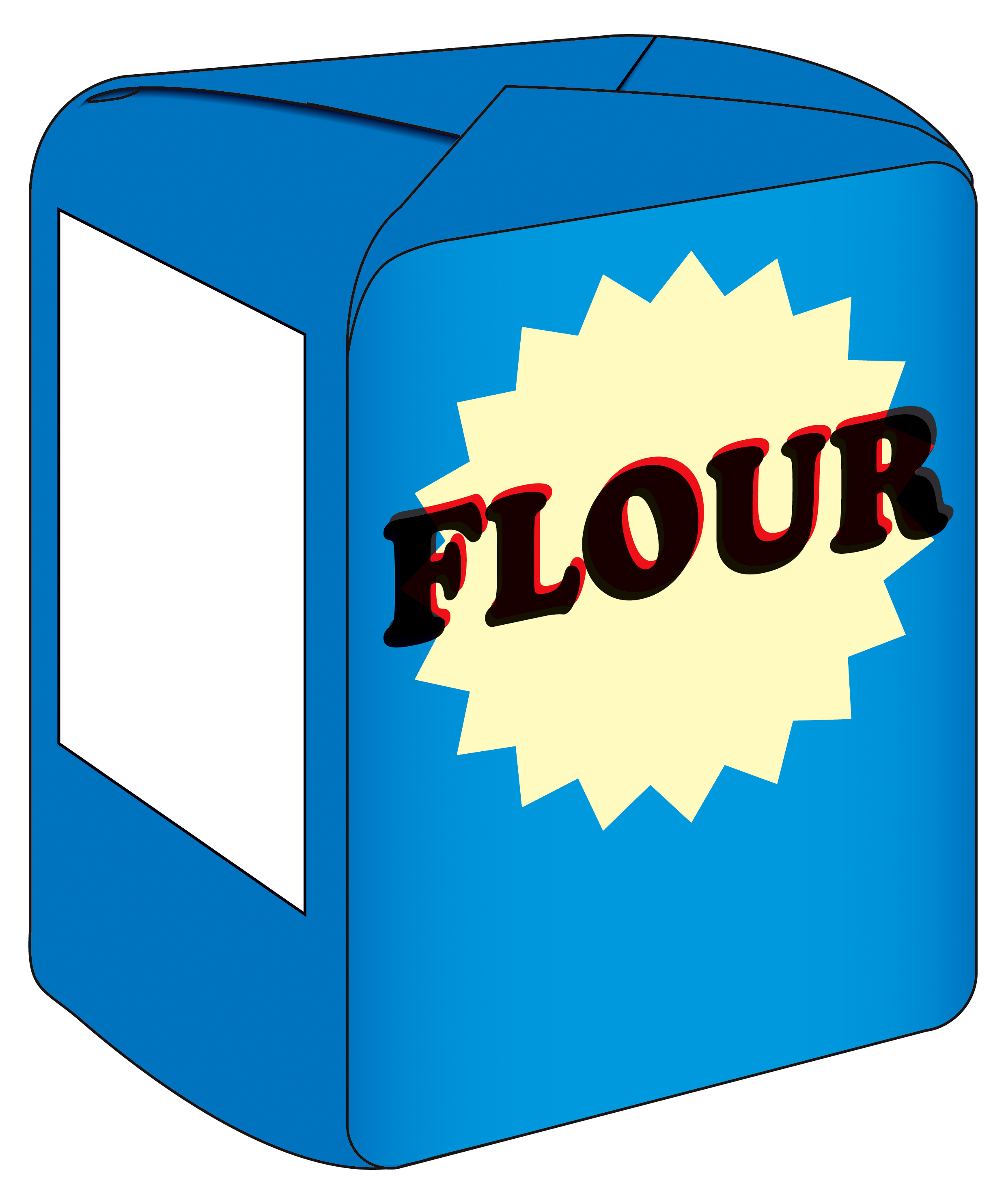Find the difference in volume between the bag of flour and the can of drink.

Flour -  base 5 cm, width 5 cm, height 8 cm

Can - radius 4 cm height 7 cm

151.90 cm³

351.90 cm³

200.90 cm³

251.90 cm³You have been asked to make eight cups of tea.

The dimensions of the mugs are radius 2.5cm, length 6 cm.

What is the total volume of liquid the mugs hold?

151.90 cm³

351.90 cm³

200.90 cm³

251.90 cm³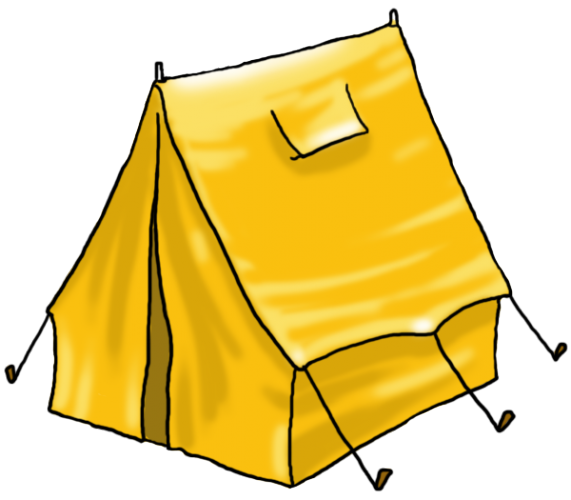This tent is 1.5 m wide, 2 m high and 3 m long.

Unfortunately, it rained and half of it filled up with water!

What is the volume of water in the tent?

151.90 cm³

351.90 cm³

200.90 cm³

251.90 cm³An animal trough is in the shape of a semi-circular prism as shown.

What is the volume of food when the trough is full to the top?

Length = 180  cm  diameter  60 cm

509450 cm³

509004 cm³

254502 cm³

28278 cm³The volume of this cake box is 960 cm³.

How many cakes in the shape of rectangular cuboids of 8 cm x 5 cm x 3 cm will fit inside?

What would you rather?

 Yes No Toblerone base 4cm height 5 cm length 7 Square chocolate box side 4 cm
• Question 1The length of this cylinder is 12 cm.  The radius of the face is 5 cm.

What is the volume of the cylinder?

942.6 cm³
EDDIE SAYS
This is where you have to stop and scratch your head to select the correct formula to find the area of the face. Here we had to find the area of the circle first. 3.142 x 5 x 5 = 78.55 cm Now multiply by the length 78.55 x 12 =942.6 cm³
• Question 2What is the volume of this triangular prism.

Base is 6 cm, height 7 cm and length 9 cm.

EDDIE SAYS
This time we need to find the area of the triangle first. 6 x 7 = 42 ÷ 2 = 21 Now just multiply by the length 21 x 9 = 189 cm³ I love Toblerone, don't you?
• Question 3

Can you work out the following?

## Column B

Cube side 8 cm
512 cm³
Triangular prism base 3cm, height...
72 cm³
Cylinder length 12 cm, radius 5 c...
942.6 cm³
Cereal box base 12 cm, width 4 cm...
720 cm³
EDDIE SAYS
A lot to remember here isn't there. Once you have the formulas for the areas of the faces sorted out you just use the length to complete finding the volume. Cube = 8 x 8 x 8 Triangular prism 3 x 6 ÷ 2 = 9 x 8 Cylinder 3.142 x 5 x 5 = 78.55 x 12 Cereal box12 x 4 x 15 You will be seeing these shapes in your sleep!
• Question 4This mouse has started eating this wedge of cheese.

The dimensions of the cheese are base 7 cm, height 8 cm and height 8 cm.

What is the volume of cheese the mouse has to get through?

EDDIE SAYS
That mouse must think it is his birthday 7 x 8 ÷ 2 = 28 cm 28 x 8 = 224cm³
• Question 5Find the difference in volume between the bag of flour and the can of drink.

Flour -  base 5 cm, width 5 cm, height 8 cm

Can - radius 4 cm height 7 cm

151.90 cm³
EDDIE SAYS
A bit of a pick and mix question! This is why it is good to know your formulas. Flour = 5 x 5 x 8 = 200 cm³ Can 3.142 x 4 x 4 = 50.272 cm x 7 = 351.90 cm³ To find the difference subtract 200 from 351.90
• Question 6You have been asked to make eight cups of tea.

The dimensions of the mugs are radius 2.5cm, length 6 cm.

What is the total volume of liquid the mugs hold?

EDDIE SAYS
3.142 x 2.5 x 2.5 x 6 = 117.825 Now just multiply up by 8, 117.825 x 8 = 942.6 cm³ Someone likes tea!!
• Question 7This tent is 1.5 m wide, 2 m high and 3 m long.

Unfortunately, it rained and half of it filled up with water!

What is the volume of water in the tent?

EDDIE SAYS
Don't you just love it when that happens? There is nothing worse that a soggy tent. 1.5 x 2 & divide; 2 = 1.5 1.5 x 3 = 4.5 m³ 4.5 ÷ 2 = 2.25 m³
• Question 8An animal trough is in the shape of a semi-circular prism as shown.

What is the volume of food when the trough is full to the top?

Length = 180  cm  diameter  60 cm

254502 cm³
EDDIE SAYS
Whoo... where did that come from? Don't panic! What do we know? We know the full prism is circular shaped. That makes life easier. find the volume of a cylinder. and halve it. HOWEVER, you were given a diameter of the trough. Radius is half the diameter = 30 cm. Now you are ready to go. 3.142 x 30 x 30 = 28278 x 180 = 509004 cm³ Now divide by 2 to get half the trough 509004 ÷ 2 = 254502 cm³
• Question 9The volume of this cake box is 960 cm³.

How many cakes in the shape of rectangular cuboids of 8 cm x 5 cm x 3 cm will fit inside?

8
EDDIE SAYS
This isn't so bad. You already know the volume of the crate. Just work out the volume of the cuboids and see how many will fit inside. 8 x 5 x 3 = 120 cm³ 960 ÷ 120 = 8 No eating the cakes, you have to pack them in the box!
• Question 10

What would you rather?

 Yes No Toblerone base 4cm height 5 cm length 7 Square chocolate box side 4 cm
EDDIE SAYS
You know the drill by now. I bet it took you seconds to get this one. The toblerone of course 4 x 5 = 20 ÷ 2 = 10 x 7 = 70 cm³ of chocolate Versus 4 x 4 x 4 = 64 cm³ ...actually I want both!
---- OR ----

Sign up for a £1 trial so you can track and measure your child's progress on this activity.

### What is EdPlace?

We're your National Curriculum aligned online education content provider helping each child succeed in English, maths and science from year 1 to GCSE. With an EdPlace account you’ll be able to track and measure progress, helping each child achieve their best. We build confidence and attainment by personalising each child’s learning at a level that suits them.

Get started